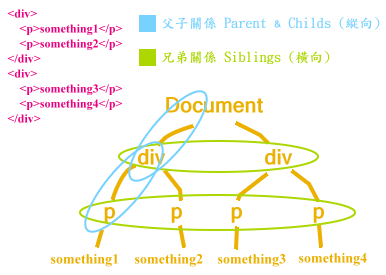# jQuery 篩選元素 (Traversing)

Traversing 也就是從某元素進一步去找出跟它有關係(關連)的元素。

## 過濾元素 (Filtering)

jQuery 有提供一些函數幫助我們方便的「濾出」我們要的目標元素：

``````.eq( index )
``````

``````\$('p').eq(2);
``````

``````.filter(selector)
``````

``````\$('p').filter('.highlight');
``````

``````.not(selector)
``````

``````\$('p').not('.green, #blueone');
``````

## 元素 (節點) 間位置的相互關係### 依縱向關係來查訪 (Finding)

``````// 取得上一階層的父元素
.parent([selector])

// 取得全部的父元素集合 (祖先元素)
.parents([selector])

// 取得(僅)下一階層的所有子元素之集合 (不含 text nodes)
.children([selector])

// 取得全部的子元素 (含 text nodes)
// 也可以用來取得 iframe 的 content document
.contents()
``````

``````// 將 li 的父元素 (可能是 <ul> 或 <ol>) 背景改為紅色
\$('li').parent().css('background-color', 'red');
// 將 li 的所有祖先元素背景都改為紅色 (直到 <html> 元素)
\$('li').parents().css('background-color', 'red');
// 將 li 的所有 <p> 祖先元素背景都改為紅色
\$('li').parents('p').css('background-color', 'red');

// 將有 .selected class 的 div 所有子元素顏色改為藍色
\$('div').children('.selected').css('color', 'blue');
``````

### 依橫向關係來查訪 (Finding)

``````// selector 用來過濾，如果我們只要符合條件的元素

// 取得其後緊鄰的兄弟元素 (同輩元素)
.next([selector])

// 取得從下一個直到最後一個同輩元素
.nextAll([selector])

// 前一個同輩元素
.prev([selector])

// 從前一個直到最開頭的同輩元素
.prevAll([selector])

// 取得其所有同輩元素的集合
.siblings([selector])
``````

### 依表達式條件來查訪元素 (Finding)

``````.find(selector)
``````
`.find()``.children()` 有點像，差別在 .children() 只會找下一層的元素而已。

``````// HTML
<p><span>Hello</span> World</p>

// jQuery
\$('p').find('span');
// 得到的結果
[<span>Hello</span>]
``````

``````\$(selector, context)

// 同上面的例子，也可以這樣寫
\$('span', \$('p'));
``````